### THE LIMIT DEFINITION OF A DEFINITE INTEGRAL

The following problems involve the limit definition of the definite integral of a continuous function of one variable on a closed, bounded interval. Begin with a continuous functionon the interval. Let...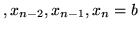be an arbitrary (randomly selected) partition of the interval, which divides the interval intosubintervals (subdivisions). Let...be the sampling numbers (or sampling points) selected from the subintervals. That is,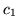is in,is in,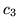is in, ... ,is in,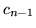is in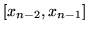,

andis in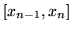.

Define the mesh of the partition to be the length of the largest subinterval. That is, letfor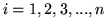and define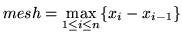.

The definite integral ofon the intervalis most generally defined to be.

For convenience of computation, a special case of the above definition usessubintervals of equal length and sampling points chosen to be the right-hand endpoints of the subintervals. Thus, each subinterval has length

equation (*)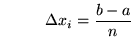forand the right-hand endpoint formula is

equation (**)for. The definite integral ofon the intervalcan now be alternatively defined by.

We will need the following well-known summation rules.

1.(n times)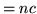, whereis a constant
2.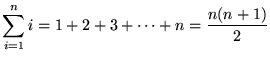3.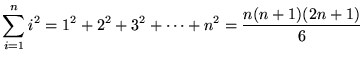4.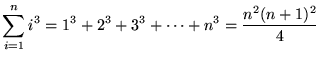5.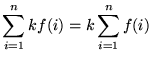, whereis a constant
6.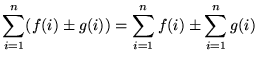Most of the following problems are average. A few are somewhat challenging. If you are going to try these problems before looking at the solutions, you can avoid common mistakes by using the formulas given above in exactly the form that they are given. Solutions to the first eight problems will use equal-sized subintervals and right-hand endpoints as sampling points as shown in equations (*) and (**) above.

• PROBLEM 1 : Use the limit definition of definite integral to evaluate.

• PROBLEM 2 : Use the limit definition of definite integral to evaluate.

• PROBLEM 3 : Use the limit definition of definite integral to evaluate.

• PROBLEM 4 : Use the limit definition of definite integral to evaluate.

• PROBLEM 5 : Use the limit definition of definite integral to evaluate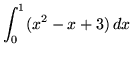.

• PROBLEM 6 : Use the limit definition of definite integral to evaluate.

• PROBLEM 7 : Use the limit definition of definite integral to evaluate.

• PROBLEM 8 : Use the limit definition of definite integral to evaluate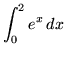.

• PROBLEM 9 : Write the following limit as a definite integral :.

• PROBLEM 10 : Write the following limit as a definite integral :.

• PROBLEM 11 : Write the following limit as a definite integral :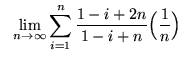.

• PROBLEM 12 : Write the following limit as a definite integral :.

• PROBLEM 13 : Write the following limit as a definite integral :.

• PROBLEM 14 : Use the limit definition of definite integral to evaluate, whereis a constant. Use an arbitrary partition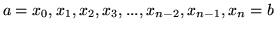and arbitrary sampling numbersfor.

• PROBLEM 15 : Use the limit definition of definite integral to evaluate. Use an arbitrary partitionand the sampling number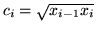for. Begin by showing thatfor. Assume that.